# 屏幕

## 屏幕空间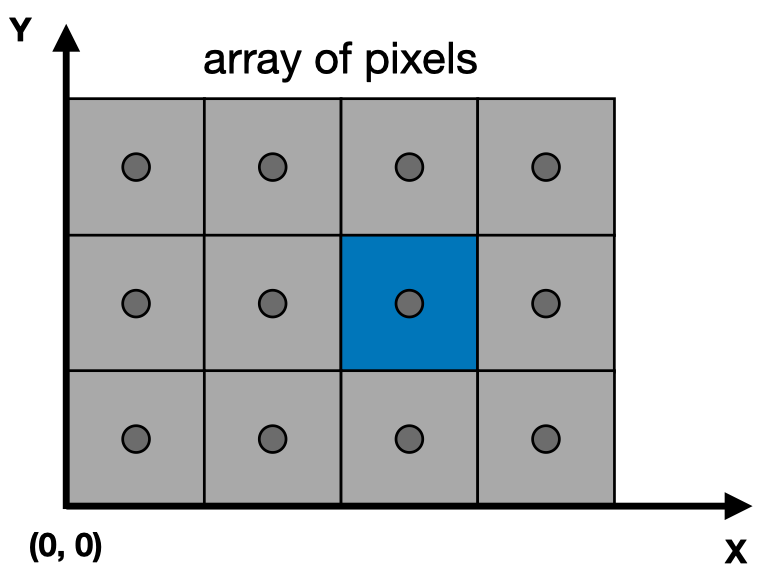• x 的取值范围是 $[0, width - 1]$
• y 的取值范围是 $[0, height - 1]$

1. 对 $[-1, 1]^2$ 进行一次缩放，铺满屏幕
2. 平移空间，使空间的左下角位于原点坐标（缩放后的空间的左下角在 $(-\frac {width} 2, -\frac {height} 2)$）

$$\begin{bmatrix} \frac {width} 2 & 0 & 0 & \frac {width} 2 \\\ 0 & \frac {height} 2 & 0 & \frac {height} 2 \\\ 0 & 0 & 1 & 0 \\\ 0 & 0 & 0 & 1 \end{bmatrix}$$

# 三角形

• 复杂图形都能通过三角形组合来表示
• 在空间中的任意三角形的三个顶点都在同一个平面内
• 能很清晰的定义出三角形的内部和外部
• 很容易计算出一个点是位于三角形内部还是外部（通过叉乘）
• 只要定义出三角形的三个顶点的属性，那三角形内部的任意一点都能通过插值的方式计算出对应的属性（通过重心坐标进行插值）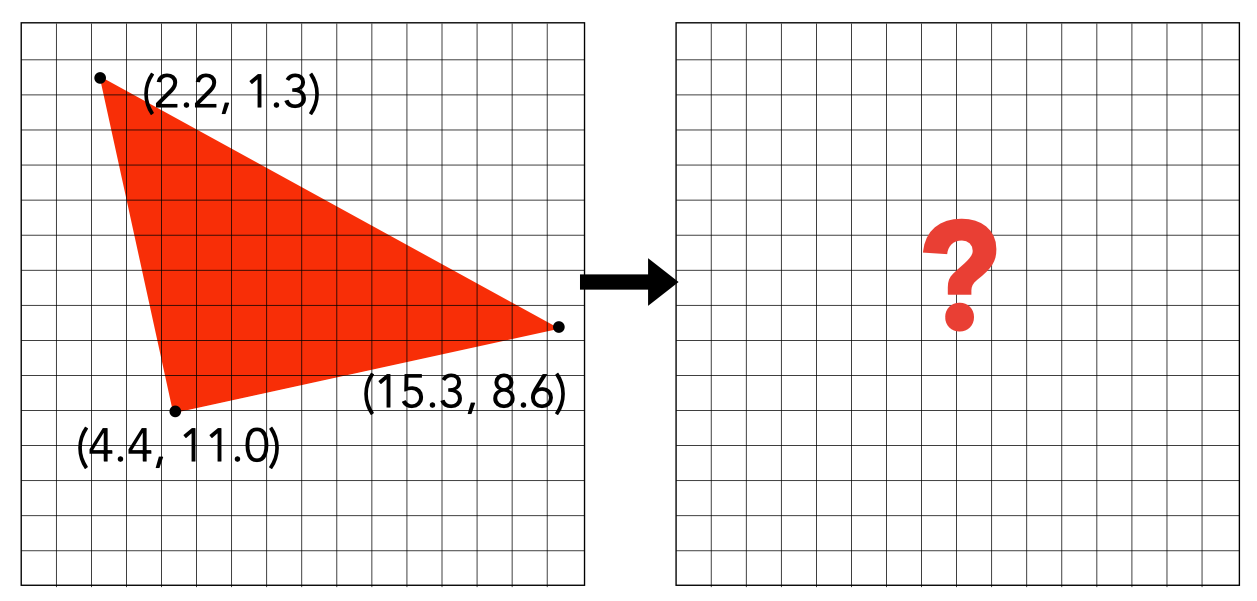# 采样

 1 2 3  for (int x = 0; x < xmax; ++x) { output[x] = f(x); }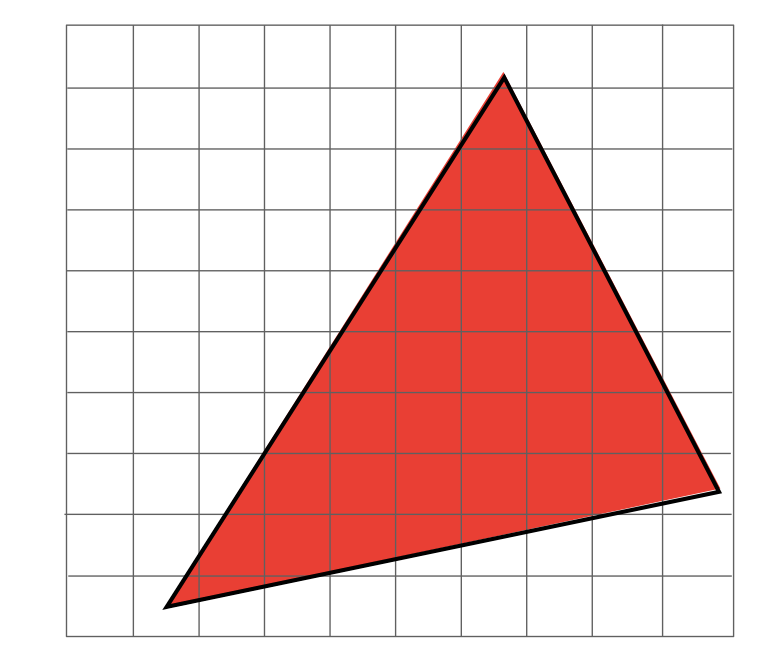$$inside(triangle, x, y) = \begin{equation} \left\{ \begin{array}{lr} 1, 当像素中心位于三角形内部时 \\ 0, 否则 \end{array} \right. \end{equation}$$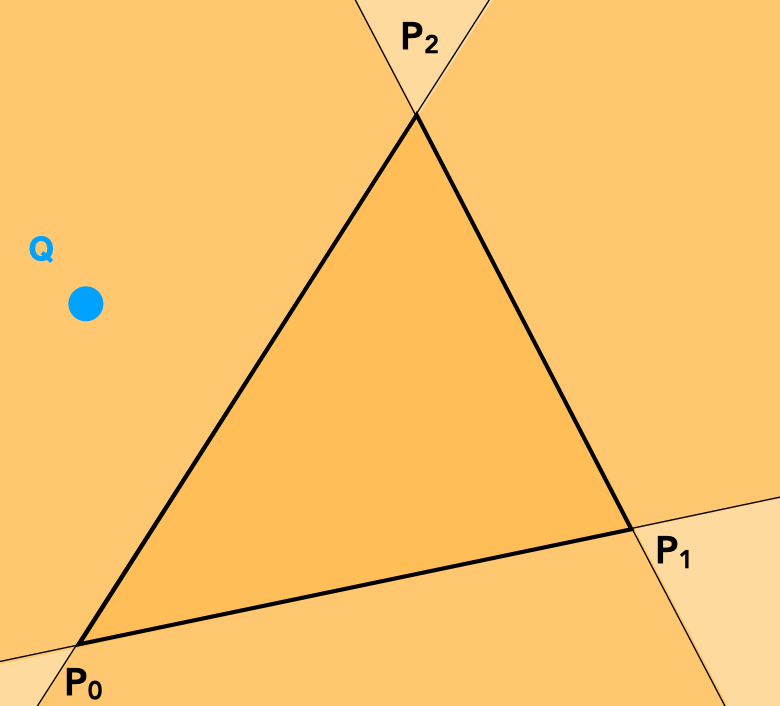$$\vec {P_0 P_1} \times \vec {P_0 Q} \\\ \vec {P_1 P_2} \times \vec {P_1 Q} \\\ \vec {P_2 P_0} \times \vec {P_2 Q} \\\$$

 1 2 3  for (int x = 0; x < width; ++x) for (int y = 0; y < height; ++y) image[x][y] = inside(triangle, x + 0.5, y + 0.5)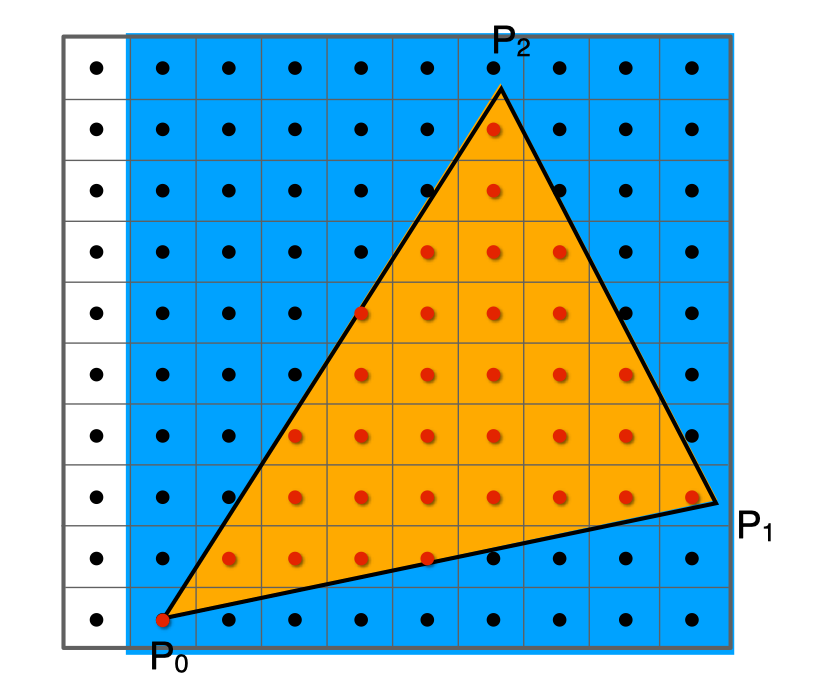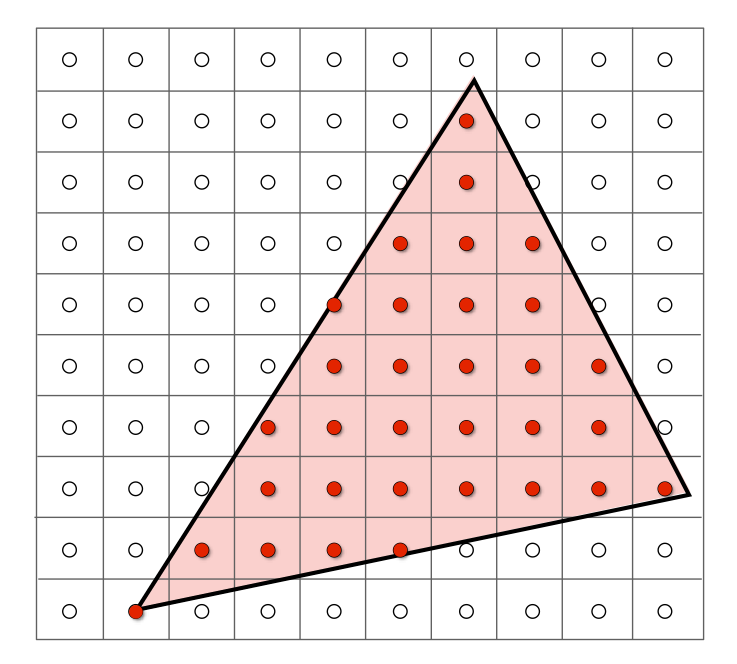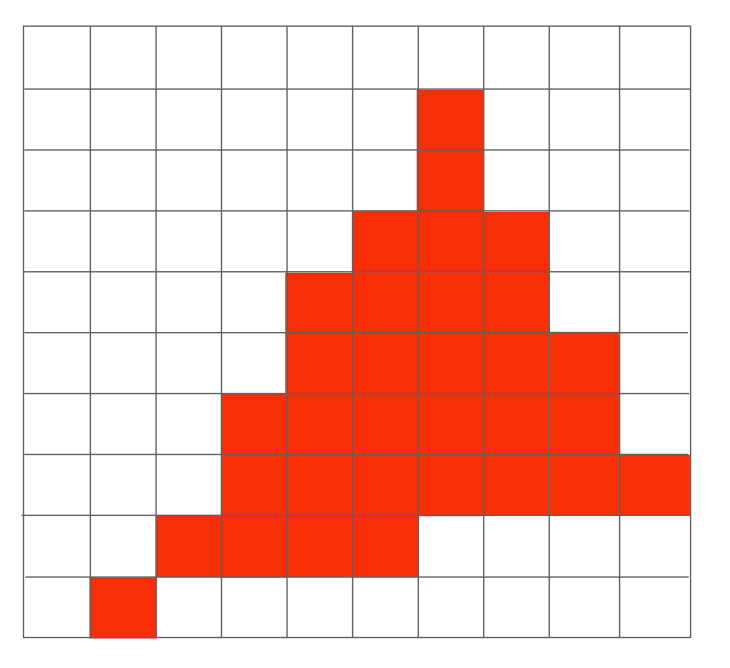# 采样理论

• 锯齿• 摩尔纹（把左图的奇数行列去掉之后，再缩放到原图尺寸就会得到右图）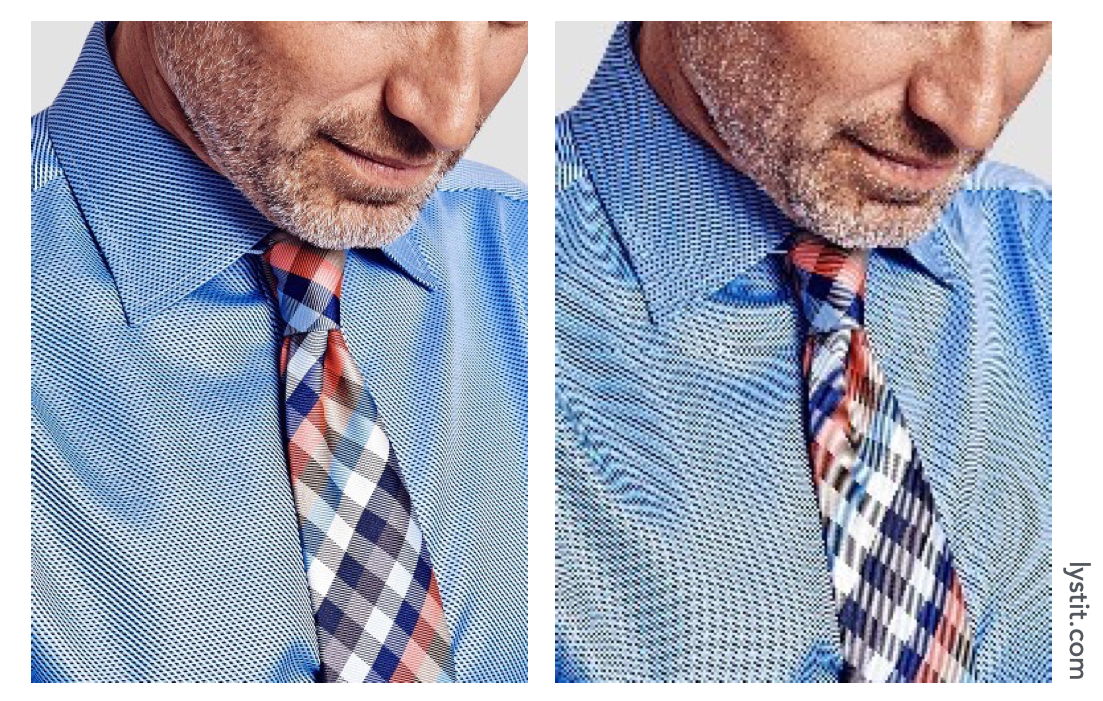• 车轮错觉（旋转得太快时感觉是往反方向旋转）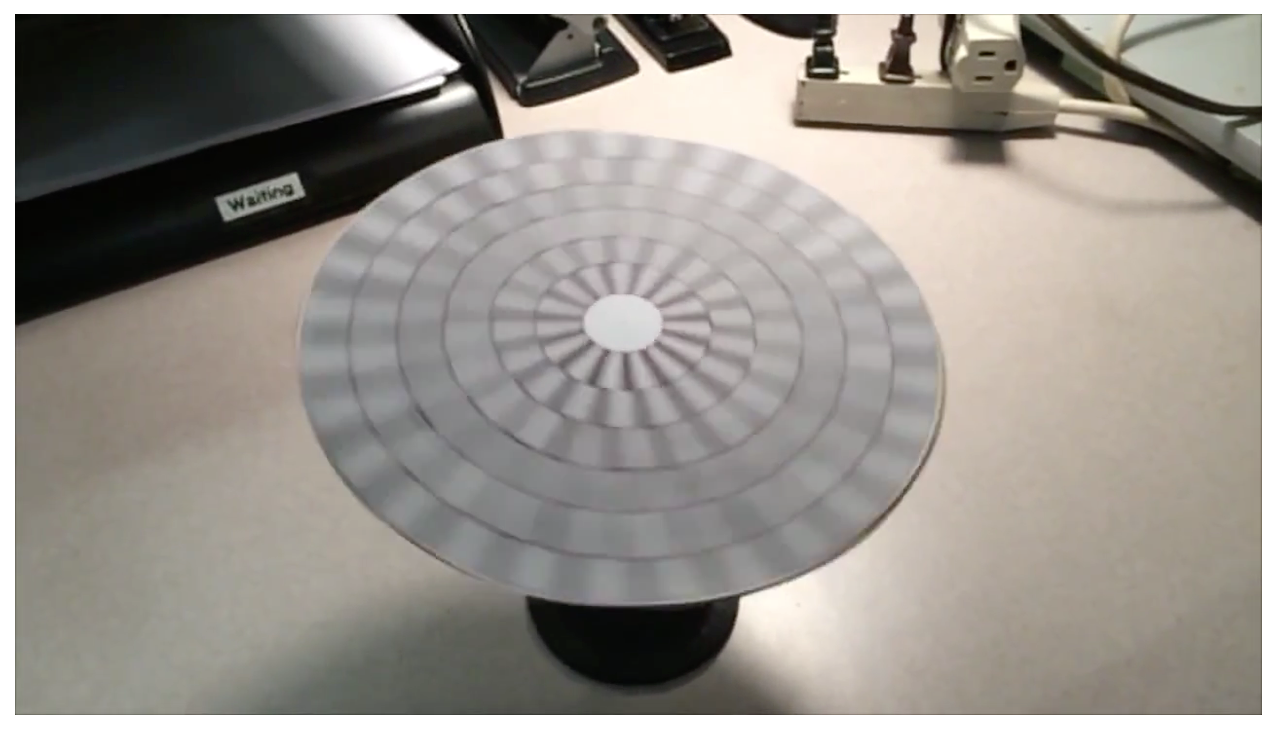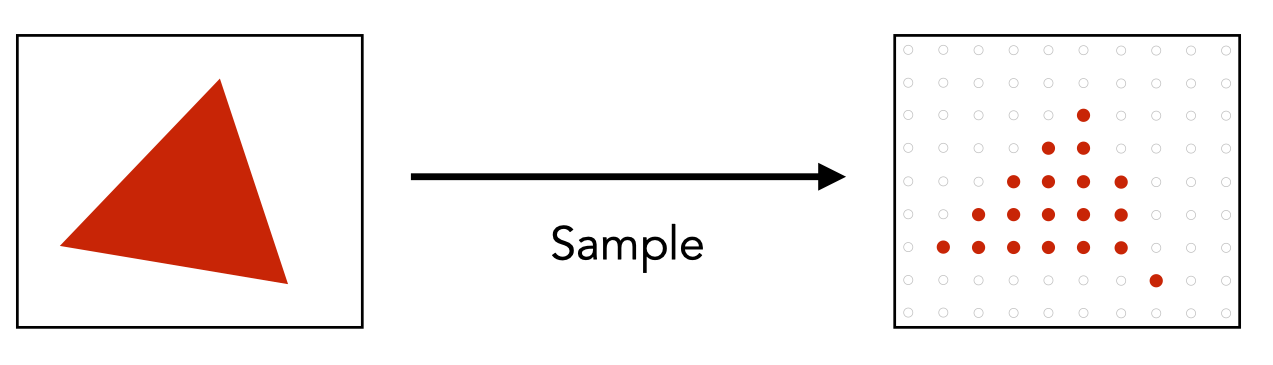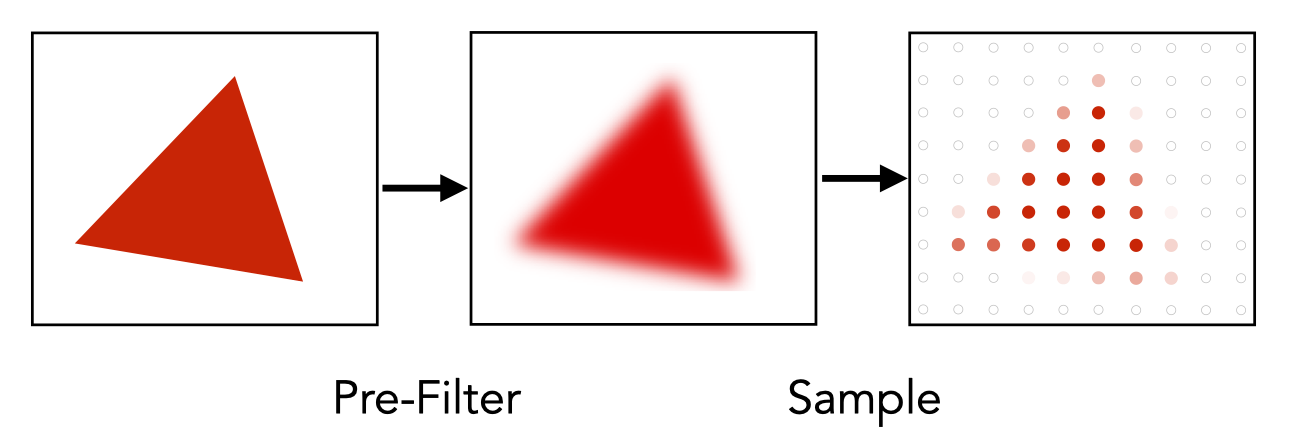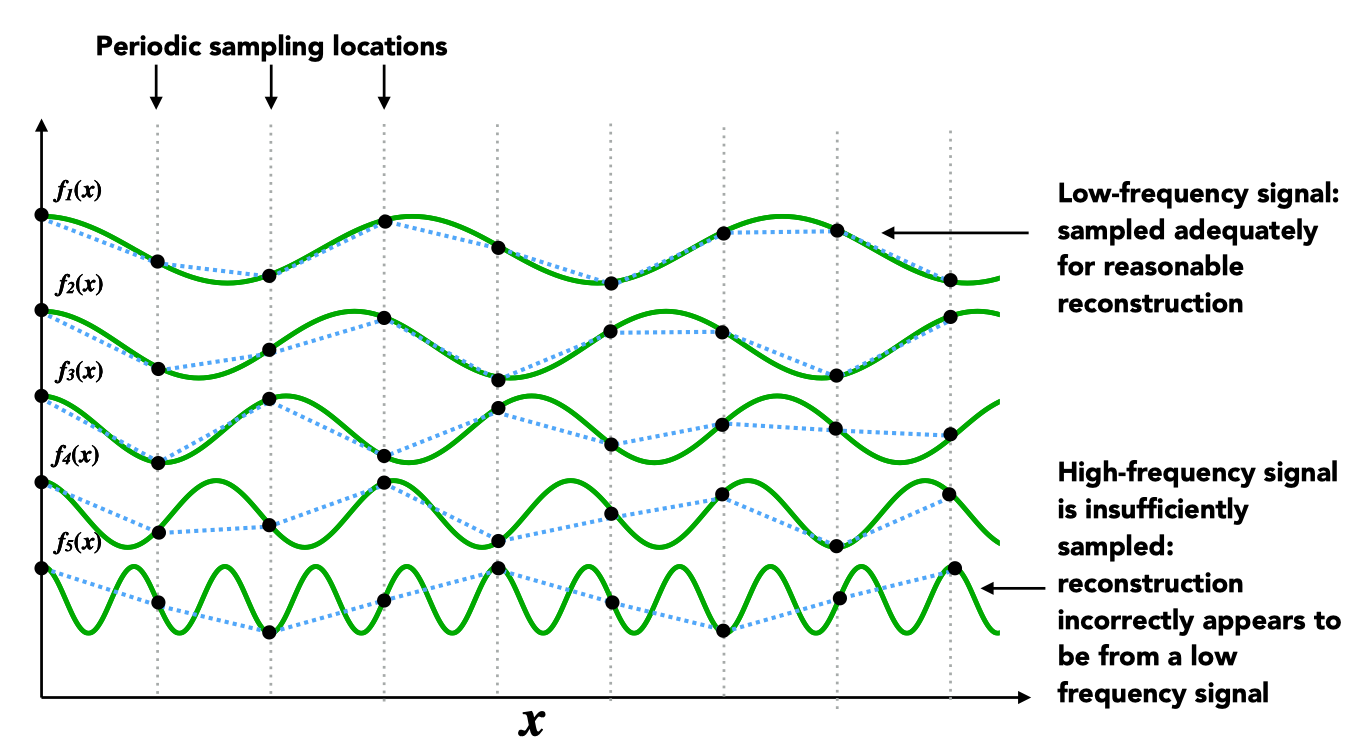• 频率越低的函数，采样后的连线与原函数差异越小
• 频率越高的函数，采样后的连线与原函数差异越大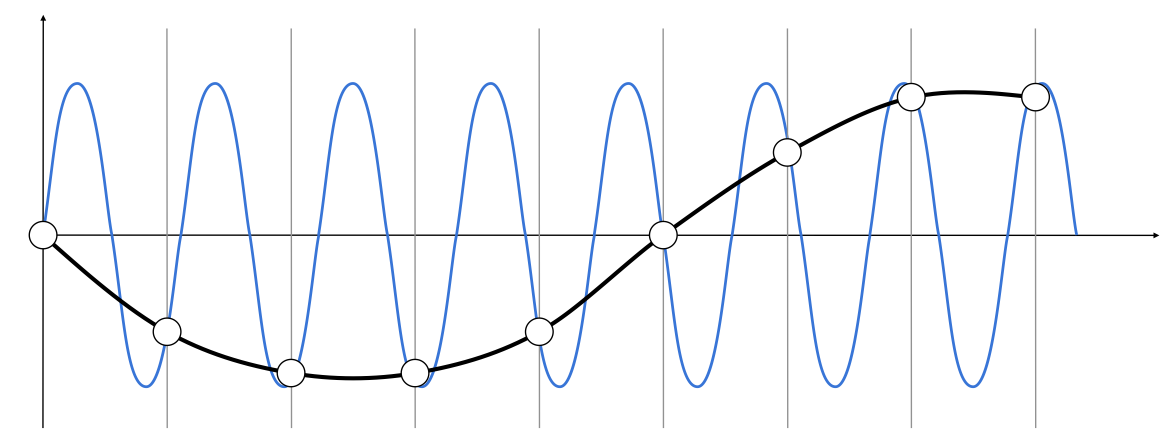# 抗锯齿

• 提高采样率

• 反走样# 超采样 （MSAA）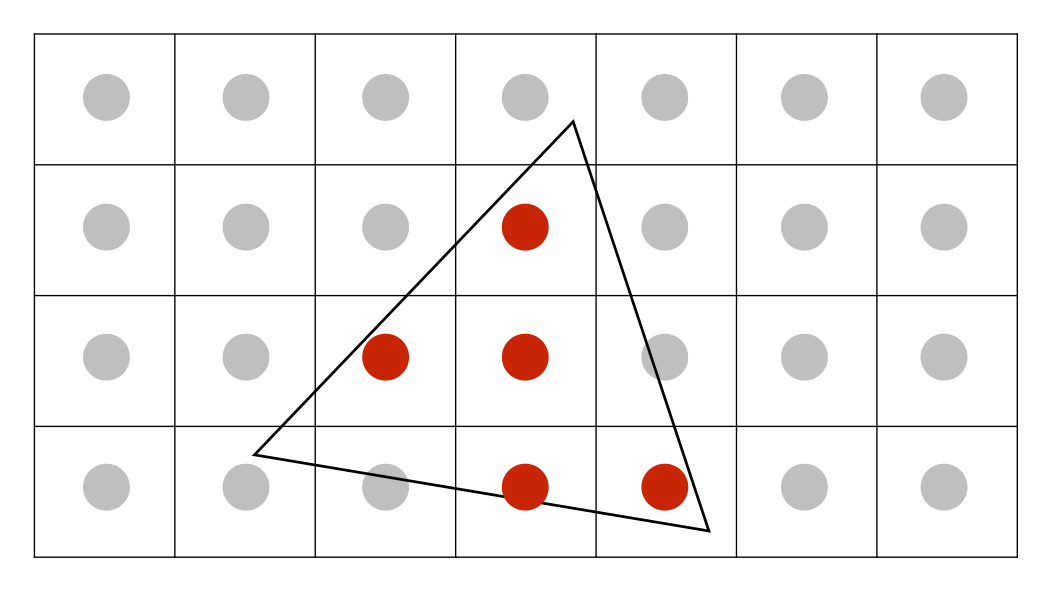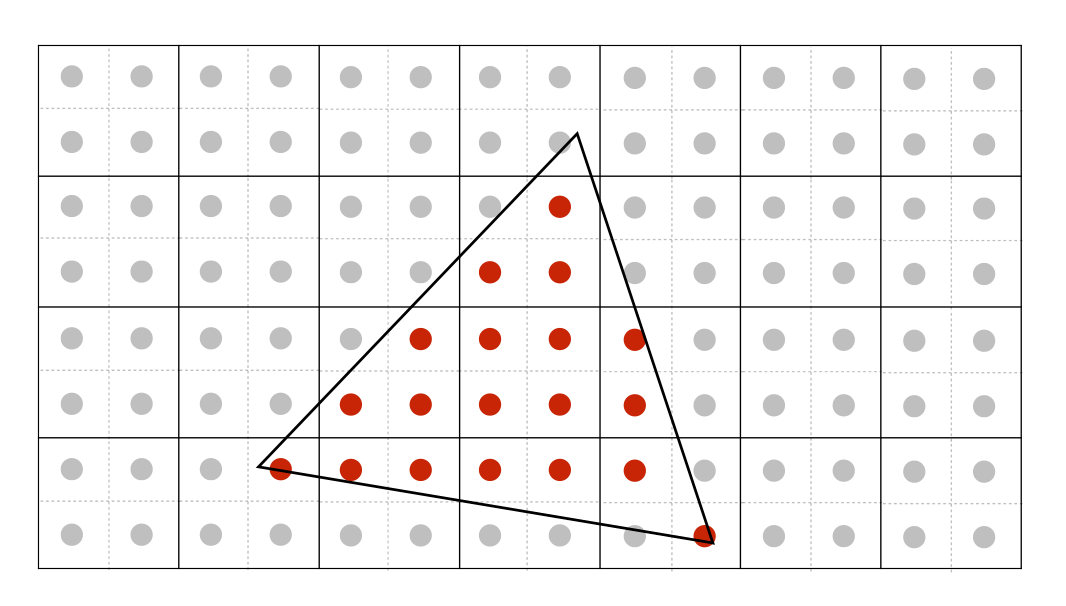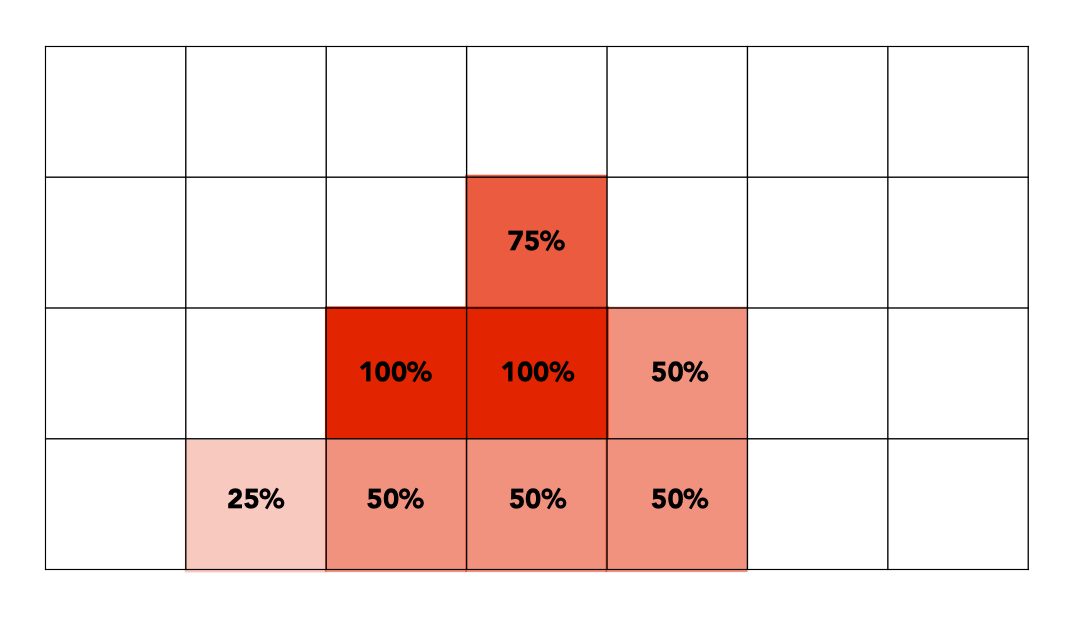• MSAA 是抗锯齿操作的第一步，也就是模糊操作（求平均）。

• MSAA 增加采样点，并不是为了提高采样率（分辨率没有提高），而是为了得到一个更合理的三角形覆盖率。

• MSAA 的代价是计算量大增，如果一个像素里面有4个采样点，那么计算量就大了 4 倍；如果一个像素里面有 16 个采样点，那么计算量就大了 16 倍。（事实上，工业界会会复用、优化这些采样点，因此计算量并没有增加太多）。

# 画家算法# 深度缓冲 （Z-Buffer）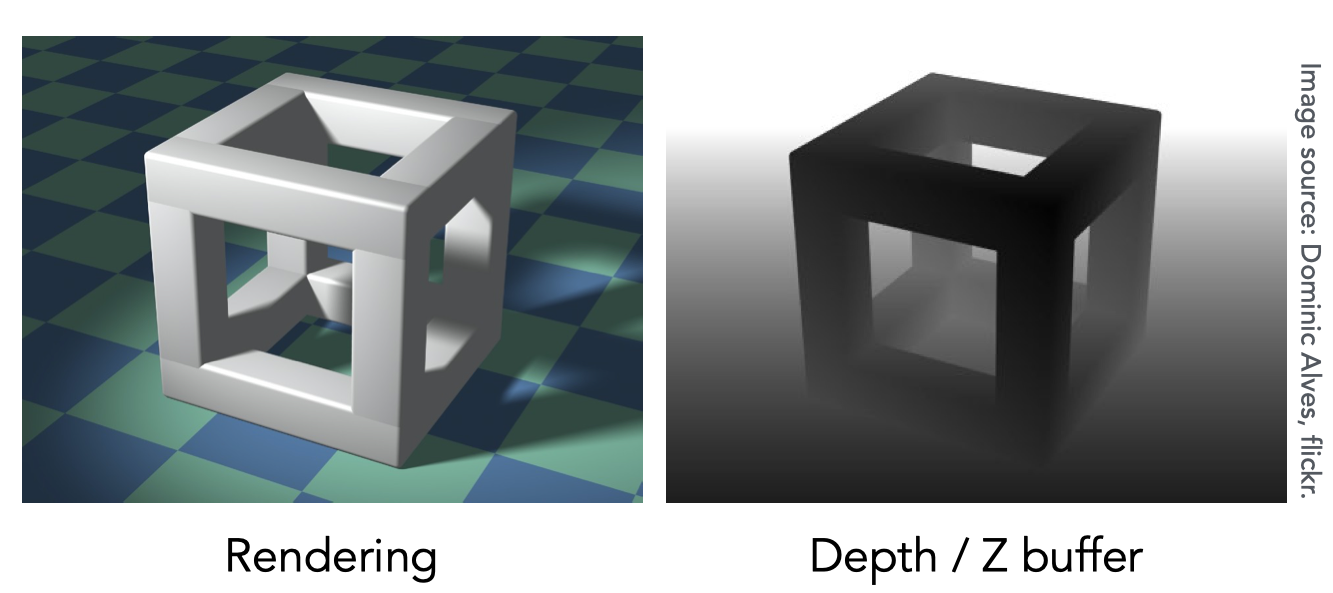1. 初始化所有像素的深度为 $\infty$
2. 在光栅化过程中，不断更新其深度：

 1 2 3 4 5 6  for (each triangle T) for (each sample(x,y,z) in T) if (z < zbuffer[x,y]) { framebuffer[x,y] = rgb; zbuffer[x,y] = z; }

1. 绘制三角形的顺序不影响最终结果
2. 所有 GPU 都支持深度测试

# 作业

## 基础

  1 2 3 4 5 6 7 8 9 10 11 12 13 14 15 16 17 18 19 20 21 22 23 24 25 26 27 28 29 30 31 32 33 34 35 36 37 38 39 40 41 42 43 44 45 46 47 48 49 50  static bool insideTriangle(int x, int y, const Vector3f* _v) { Eigen::Vector3f p0 = _v; Eigen::Vector3f p1 = _v; Eigen::Vector3f p2 = _v; Eigen::Vector3f q(x, y, 0); Eigen::Vector3f p0p1 = p1 - p0; Eigen::Vector3f p1p2 = p2 - p1; Eigen::Vector3f p2p0 = p0 - p2;; Eigen::Vector3f p0q = q - p0; Eigen::Vector3f p1q = q - p1; Eigen::Vector3f p2q = q - p2; Eigen::Vector3f r0 = p0p1.cross(p0q); Eigen::Vector3f r1 = p1p2.cross(p1q); Eigen::Vector3f r2 = p2p0.cross(p2q); return (r0.z() > 0 && r1.z() > 0 && r2.z() > 0) || (r0.z() < 0 && r1.z() < 0 && r2.z() < 0); } void rst::rasterizer::rasterize_triangle(const Triangle& t) { auto v = t.toVector4(); int top = ceil(std::max(v.y(), std::max(v.y(), v.y()))); int bottom = floor(std::min(v.y(), std::min(v.y(), v.y()))); int left = floor(std::min(v.x(), std::min(v.x(), v.x()))); int right = ceil(std::max(v.x(), std::max(v.x(), v.x()))); for (int x = left; x < right; x++) { for (int y = bottom; y < top; y++) { if (insideTriangle(x, y, t.v)) { auto[alpha, beta, gamma] = computeBarycentric2D(x, y, t.v); float w_reciprocal = 1.0/(alpha / v.w() + beta / v.w() + gamma / v.w()); float z_interpolated = alpha * v.z() / v.w() + beta * v.z() / v.w() + gamma * v.z() / v.w(); z_interpolated *= w_reciprocal; Eigen::Vector3f point(x, y, 0.0); auto ind = (height-1-point.y())*width + point.x(); if (depth_buf[ind] > z_interpolated) { depth_buf[ind] = z_interpolated; set_pixel(point, t.getColor()); } } } } } 

## 提高

  1 2 3 4 5 6 7 8 9 10 11 12 13 14 15 16 17 18 19 20 21 22 23 24 25 26 27 28 29 30 31 32 33 34 35 36 37 38 39 40 41 42 43 44 45 46 47 48 49 50 51 52 53 54 55 56 57 58 59 60 61 62 63 64 65 66 67 68 69 70 71  static bool insideTriangle(float x, float y, const Vector3f* _v) { Eigen::Vector3f p0 = _v; Eigen::Vector3f p1 = _v; Eigen::Vector3f p2 = _v; Eigen::Vector3f q(x, y, 0); Eigen::Vector3f p0p1 = p1 - p0; Eigen::Vector3f p1p2 = p2 - p1; Eigen::Vector3f p2p0 = p0 - p2;; Eigen::Vector3f p0q = q - p0; Eigen::Vector3f p1q = q - p1; Eigen::Vector3f p2q = q - p2; Eigen::Vector3f r0 = p0p1.cross(p0q); Eigen::Vector3f r1 = p1p2.cross(p1q); Eigen::Vector3f r2 = p2p0.cross(p2q); return (r0.z() > 0 && r1.z() > 0 && r2.z() > 0) || (r0.z() < 0 && r1.z() < 0 && r2.z() < 0); } //Screen space rasterization void rst::rasterizer::rasterize_triangle(const Triangle& t) { auto v = t.toVector4(); int top = ceil(std::max(v.y(), std::max(v.y(), v.y()))); int bottom = floor(std::min(v.y(), std::min(v.y(), v.y()))); int left = floor(std::min(v.x(), std::min(v.x(), v.x()))); int right = ceil(std::max(v.x(), std::max(v.x(), v.x()))); float sub_pixels = { { 0.0, 0.0 }, { 0.5, 0.0 }, { 0.0, 0.5 }, { 0.5, 0.5 } }; int sub_pixels_length = sizeof(sub_pixels) / sizeof(sub_pixels); for (int x = left; x < right; x++) { for (int y = bottom; y < top; y++) { float pass = 0.0; for (int i = 0; i < sub_pixels_length; i++) { if (insideTriangle(x + sub_pixels[i], y + sub_pixels[i], t.v)) { pass += 1; } } // NOTE: percent MUST be float, make sure pass or sub_pixels_length is float. float percent = pass / sub_pixels_length; if (pass > 0) { auto[alpha, beta, gamma] = computeBarycentric2D(x, y, t.v); float w_reciprocal = 1.0/(alpha / v.w() + beta / v.w() + gamma / v.w()); float z_interpolated = alpha * v.z() / v.w() + beta * v.z() / v.w() + gamma * v.z() / v.w(); z_interpolated *= w_reciprocal; Eigen::Vector3f color = t.getColor(); Eigen::Vector3f point(x, y, 0.0); auto ind = (height-1-point.y())*width + point.x(); if (depth_buf[ind] > z_interpolated) { depth_buf[ind] = z_interpolated; color *= percent; set_pixel(point, color); } } } } } 

# 参考资料

Lecture 05 Rasterization 1 (Triangles)

Lecture 06 Rasterization 2 (Antialiasing and Z-Buffering)